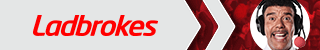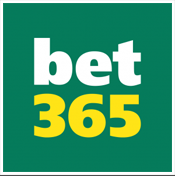# How to Read Betting Odds

Published on: 20/11/2016Depending on your location, you will see betting odds listed in different ways. You may already be familiar with one particular format; however, it is worth understanding how they all work.

The odds are basically the probability of each outcome occurring. The bookmakers calculate the odds using sophisticated software as well as manual techniques. They then build in a small commission to every price to ensure that they always have the advantage. Most odds are displayed in two ways: they either show how much you will win (return odds) or how much must be staked to win a certain amount (Money-line odds).

Fractional and decimal odds are essentially the same but displayed in a different way. Fractional odds are favoured in the UK and show the return relative to the stake.

Example:

• Odds: 6/1
• Profit: 6
• Stake unit: 1
• Total return including stake: 6 + 1 = 7

So, a €1 bet would return a €6 profit and a total return (including stake) of €7. The nature of fractions means that the stake cannot always be displayed as a single unit. So if the net return is 2.5 for every unit, the odds would show as 5/2. Where the stake and return is equal (1/1) the odds are referred to as Evens.

Decimal odds are favoured in many countries across Europe and also in Australia and Canada. They are calculated by converting the fractional odds into a decimal and adding one unit. So 6/1 would be 6 divided by 1 plus 1.

Example:

• Odds: 7.00
• Profit: 6
• Stake unit: 1
• Total return including stake: 6 + 1 = 7

As before, a €1 bet would return a €6 profit and a total return (including stake) of €7. Odds of 1/1 or Evens are displayed as 2.00. In Hong Kong, decimal odds are used but they reflect the profit not the overall return.

Example:

• Odds: 6.00
• Profit: 6
• Stake unit: 1
• Total return including stake: 6 + 1 = 7

In this case, odds of 1/1 or Evens (2.00) would appear as 1.00. Money-line odds are favoured by bookmakers in the USA. The odds can be positive or negative and display either the value of the stake required to win 100 units or the profit that can be won from a stake of 100 units.

They are calculated by multiplying fractional odds by 100. So odds of 6/1 would appear as +600.

Example:

• Odds: +600.00
• Net return: 600
• Stake unit: 100
• Total return including stake: 600 + 100 = 700

So, a €1 bet would return a €6 profit and a total return (including stake) of €7. With this format, Evens odds are displayed as +100. However, if the fraction is less than one, then the Money-line appears as a negative number. So fractional odds of 1/6 would appear as -600.

Example:

• Odds: -600.00
• Net return: 100
• Stake unit: 600
• Total return including stake: 100 + 600 = 700

In this case, a €600 bet would be required just to return a €0 profit. Therefore, a positive money line displays the profit from a 100 unit stake and a negative money-line displays the stake required to win 100 units.

Once you understand how to read the odds, you can choose the format that appeals to you most by changing the settings on your favourite betting websites.

Published on: 20/11/2016 © Bet Bind